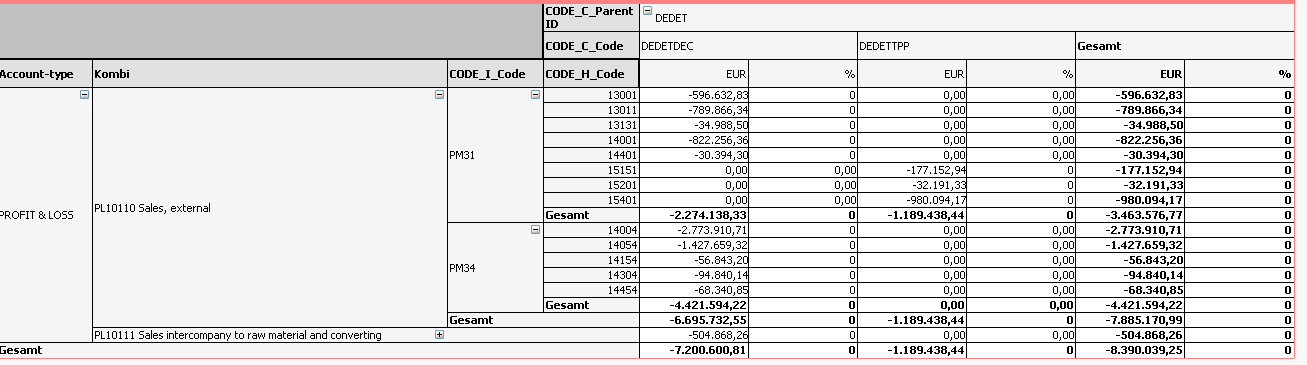# QlikView App Dev

Discussion Board for collaboration related to QlikView App Development.

cancel
Showing results for
Did you mean:Not applicable

## percentage of sales

Hi everybody,

I have a Problem and hope that somebody of you could help me.

I created a Pivot table of sales and want to see the percentage of total sales.

The Formular for the sales is very easy (sum(valuehome).

What Formular should I use to get the percentage regarding to total (i.e. 596632,83/7200600,81 =8,29%)

I would have expect that "valuehome/sum(valuehome " would work but it doesnt.

Maybe it would be also possible to define the total line (7200600,81) as Total line to calculate with that (but I dont know how).

Does somebody of you maybe have an idea?1 Solution

Accepted SolutionsSpecialist II

HiChristian

you can use if(dimensionality()=2,sum(valuehomw)/sum(total valuehomw),else condition)

4 RepliesSpecialist II

HiChristian

you can use if(dimensionality()=2,sum(valuehomw)/sum(total valuehomw),else condition)Partner

Something like:

Sum(TOTAL <CODE_I_Code> valuehome)

Logic will get you from a to b. Imagination will take you everywhere. - A EinsteinNot applicable
Author

thanks a lot. That helped.

Could you please tell me how to set up this Formular as Variable

I put into the script:

Set Var_T1_Key =(sum(Valuehome)/aggr(NODISTINCT sum(Valuehome),CODE_C_Code));

I got the Variable but it does not calculate and I dont know why.Partner

Just

Sum(valuehome )/Sum(TOTAL valuehome )

The TOTAL keyword is for disregarding dimension(s), you could even specify the fields with <Dim1,Dim2..>

Another option would be to just use Sum(valuehome) as expression and set it to relative (Checkbox)